# 當TensorFlow遇見CNTK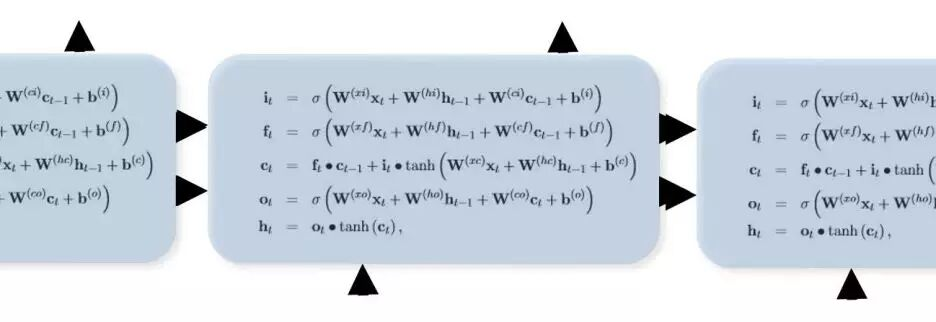CNTK是微軟用於搭建深度神經網路的計算網路工具包，此項目已在Github上開源。因為我最近寫了關於TensorFlow的文章，所以想比較一下這兩個系統的相似和差異之處。畢竟，CNTK也是許多圖像識別挑戰賽的衛冕冠軍。為了內容的完整性，我應該也對比一下Theano、Torch和Caffe。後三者也是現在非常流行的框架。但是本文僅限於討論CNTK和TensorFlow，其餘的框架將在今後討論。Kenneth Tran對這五個深度學習工具包做過一次高水平（以他個人觀點）的分析。本文並不是一個CNTK或者TensorFlow的使用教程。我的目的在於從工程師的角度對它們做高層次的對比。本文也不屬於性能分析，而是編程模型分析。文中會夾雜著大量的代碼，如果你討厭閱讀代碼，請直接跳到結論部分。

CNTK有一套極度優化的運行系統來訓練和測試神經網路，它是以抽象的計算圖形式構建。如此看來，CNTK和TensorFlow長得非常相似。但是，它們有一些本質上的區別。為了演示這些特性和區別，我會用到兩個標準示例，它們分別包括了兩個系統及調用各自系統完成的任務。第一個例子是用較淺的卷積神經網路來解決標準的MNIST手寫數字集的識別任務。我會針對它們兩種遞歸神經網路方法的差異性做一些點評總結。

TensorFlow和CNTK都屬於腳本驅動型的。我的意思是說神經網路構建的流程圖都是在一個腳本裡完成，並調用一些智能的自動化步驟完成訓練。TensorFlow的腳本是與Python語言捆綁的，Python操作符能夠用來控制計算圖的執行過程。CNTK目前還沒有和Python或是C++綁定（盡管已經承諾過），所以它目前訓練和測試的流程控制還是需要精心編制設計的。等會我將展示，這個過程並不能算是一種限制。CNTK網路需要用到兩個腳本：一個控制訓練和測試參數的配置文件和一個用於構建網路的網路定義語言（Network Definition Language, NDL）文件。

CNTK網路圖有一些特殊的節點。它們是描述輸入數據和訓練標籤的FeatureNodes和LabelNodes，用來評估訓練結果的CriterionNodes和EvalNodes，和表示輸出的OutputNodes。當我們在下文中遇到它們的時候我再具體解釋。在文件頂部還有一些用來加載數據（特徵）和標籤的宏定義。如下所示，我們將MNIST數據集的圖像作為特徵讀入，經過歸一化之後轉化為若干浮點數組。得到的數組「featScaled」將作為神經網路的輸入值。

# the actual NDL that defines the network
run = DNN
ndlMnistMacros = [
imageW = 28, imageH = 28
labelDim = 10
features = ImageInput(imageW, imageH, 1)
featScale = Const(0.00390625)
featScaled = Scale(featScale, features)
labels = Input(labelDim)
]
DNN=[
# conv1
kW1 = 5, kH1 = 5
cMap1 = 16
hStride1 = 1, vStride1 = 1
conv1_act = ConvReLULayer(featScaled,cMap1,25,kW1,kH1,hStride1,vStride1,10, 1)
# pool1
pool1W = 2, pool1H = 2
pool1hStride = 2, pool1vStride = 2
pool1 = MaxPooling(conv1_act, pool1W, pool1H, pool1hStride, pool1vStride)
# conv2
kW2 = 5, kH2 = 5
cMap2 = 32
hStride2 = 1, vStride2 = 1
conv2_act = ConvReLULayer(pool1,cMap2,400,kW2, kH2, hStride2, vStride2,10, 1)
# pool2
pool2W = 2, pool2H = 2
pool2hStride = 2,  pool2vStride = 2
pool2 = MaxPooling(conv2_act, pool2W, pool2H, pool2hStride, pool2vStride)
h1Dim = 128
h1 = DNNSigmoidLayer(512, h1Dim, pool2, 1)
ol = DNNLayer(h1Dim, labelDim, h1, 1)
ce = CrossEntropyWithSoftmax(labels, ol)
err = ErrorPrediction(labels, ol)
# Special Nodes
FeatureNodes = (features)
LabelNodes = (labels)
CriterionNodes = (ce)
EvalNodes = (err)
OutputNodes = (ol)
]

DNN小節定義了網路的結構。此神經網路包括了兩個卷積-最大池化層，接著是有一個128節點隱藏層的全連接標準網路。

ConvReLULayer的NDL代碼如下圖所示：
ConvReLULayer(inp, outMap, inWCount, kW, kH, hStride, vStride, wScale, bValue) =
[
convW = Parameter(outMap, inWCount, init=”uniform”, initValueScale=wScale)
convB = Parameter(outMap, 1,        init=”fixedValue”, value=bValue)
conv = Convolution(convW, inp, kW, kH, outMap, hStride,vStride,
convPlusB = Plus(conv, convB);
act = RectifiedLinear(convPlusB);
]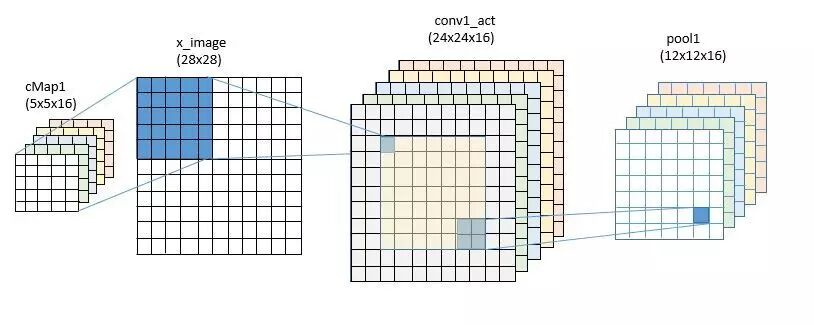DNNSigmoidLayer(inDim, outDim, x, parmScale) = [
W = Parameter(outDim, inDim, init=”uniform”, initValueScale=parmScale)
b = Parameter(outDim, 1,     init=”uniform”, initValueScale=parmScale)
t = Times(W, x)
z = Plus(t, b)
y = Sigmoid(z)
]
DNNLayer(inDim, outDim, x, parmScale) = [
W = Parameter(outDim, inDim, init=”uniform”, initValueScale=parmScale)
b = Parameter(outDim, 1,     init=”uniform”, initValueScale=parmScale)
t = Times(W, x)
z = Plus(t, b)
]

def weight_variable(shape, names):
initial = tf.truncated_normal(shape, stddev=0.1)
return tf.Variable(initial, name=names)
def bias_variable(shape, names):
initial = tf.constant(0.1, shape=shape)
return tf.Variable(initial, name=names)
x = tf.placeholder(tf.float32, [None, 784], name=”x”)
sess = tf.InteractiveSession()
W_conv1 = weight_variable([5, 5, 1, 32], “wconv”)
b_conv1 = bias_variable(, “bconv”)
W_conv2 = weight_variable([5, 5, 32, 64], “wconv2”)
b_conv2 = bias_variable(, “bconv2”)
W_fc1 = weight_variable([7 * 7 * 64, 1024], “wfc1”)
b_fc1 = bias_variable(, “bfcl”)
W_fc2 = weight_variable([1024, 10], “wfc2”)
b_fc2 = bias_variable(, “bfc2”)

def conv2d(x, W):
return tf.nn.conv2d(x, W, strides=[1, 1, 1, 1], padding='SAME')
def max_pool_2x2(x):
return tf.nn.max_pool(x, ksize=[1, 2, 2, 1],
#first convolutional layer
x_image = tf.reshape(x, [-1,28,28,1])
h_conv1 = tf.nn.relu(conv2d(x_image, W_conv1) + b_conv1)
h_pool1 = max_pool_2x2(h_conv1)
#second convolutional layer
h_conv2 = tf.nn.relu(conv2d(h_pool1, W_conv2) + b_conv2)
h_pool2 = max_pool_2x2(h_conv2)
#final layer
h_pool2_flat = tf.reshape(h_pool2, [-1, 7*7*64])
h_fc1 = tf.nn.relu(tf.matmul(h_pool2_flat, W_fc1) + b_fc1)
h_fc1_drop = tf.nn.dropout(h_fc1, keep_prob)
y_conv=tf.nn.softmax(tf.matmul(h_fc1_drop, W_fc2) + b_fc2)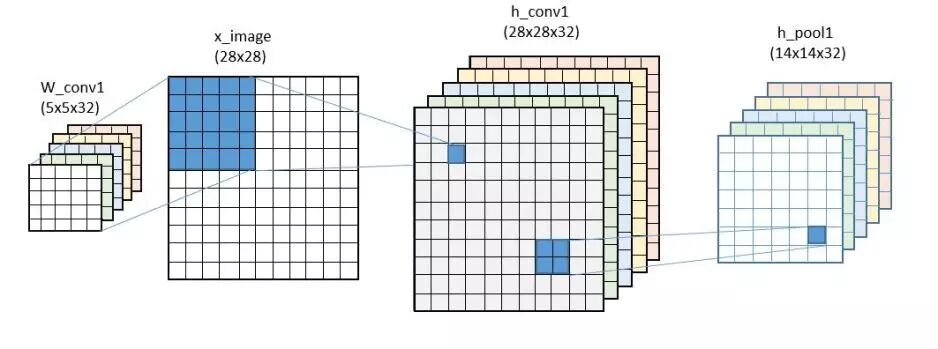## 網路訓練

CNTK中設置網路模型訓練的方式與TensorFlow差別巨大。訓練和測試步驟是在一個convolution.config的文件內設置。CNTK和TensorFlow都是通過符號化分析流程圖來計算梯度下降訓練算法中所用到的梯度值。CNTK組給出了一本非常讚的「書」來闡述梯度是如何計算的。現階段CNTK只支持一種學習方法：Mini-batch隨機梯度下降法，但他們承諾今後加入更多的算法。He, Zhang, Ren 和 Sun發表了一篇優秀的論文介紹他們是如何用嵌套殘留還原法（nested residual reduction）來訓練極度深層（深達1000層）的網路模型，所以讓我們拭目以待這個方法被融入到CNTK中。配置文件的縮略版如下所示。

command = train:test
modelPath = “$ModelDir$/02_Convolution”
ndlMacros = “$ConfigDir$/Macros.ndl”
train = [
action = “train”
NDLNetworkBuilder = [
networkDescription = “$ConfigDir$/02_Convolution.ndl”
]
SGD = [
epochSize = 60000
minibatchSize = 32
learningRatesPerMB = 0.5
momentumPerMB = 0*10:0.7
maxEpochs = 15
]
file = “$DataDir$/Train-28×28.txt”
features = [
dim = 784
start = 1
]
labels = [
# details deleted
]
]
]
test = [
….
]

16核（沒有GPU）的Linux VM需要消耗62.95分鐘來執行訓練和測試過程，999.01分鐘的用戶時間和4分鐘的系統時間。用戶時間指的是所有16個核都在滿負荷運轉(999/63 = 15.85)。但這並不算什麼，因為CNTK是為並行計算而設計的，大規模GPU支持才是真正的設計點。

y_ = tf.placeholder(tf.float32, [None, 10])
cross_entropy = -tf.reduce_sum(y_*tf.log(y_conv))
correct_prediction = tf.equal(tf.argmax(y_conv,1), tf.argmax(y_,1))
accuracy = tf.reduce_mean(tf.cast(correct_prediction, “float”))
sess.run(tf.initialize_all_variables())
for i in range(20000):
batch = mnist.train.next_batch(50)
train_step.run(feed_dict={x: batch, y_: batch, keep_prob: 0.5})
print(“test accuracy %g”%accuracy.eval(feed_dict={
x: mnist.test.images, y_: mnist.test.labels, keep_prob: 1.0}))

## 遞歸神經網路在CNTK和TensorFlow的做到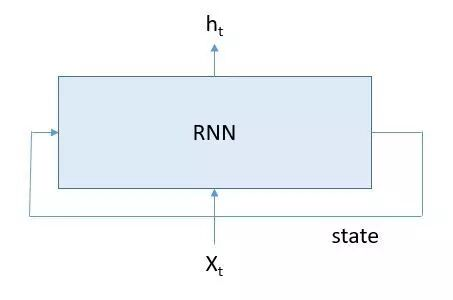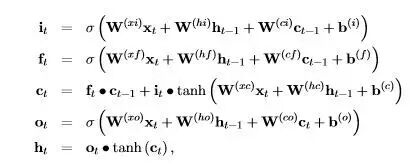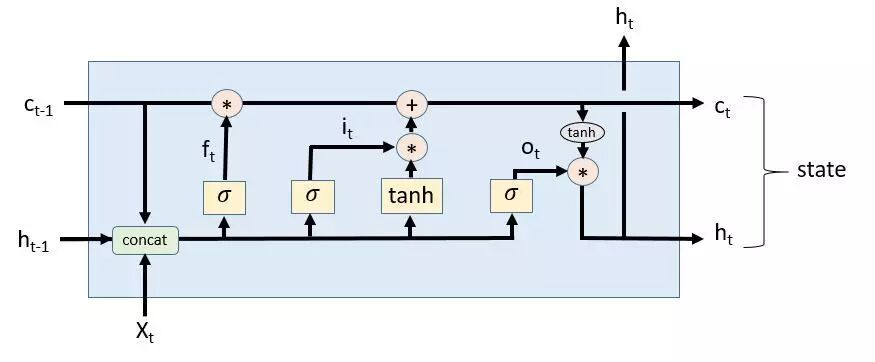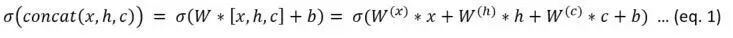### CNTK版本

LSTMPComponent(inputDim, outputDim, cellDim, inputx, cellDimX2, cellDimX3, cellDimX4) = [
wx = Parameter(cellDimX4, inputDim,  init=”uniform”, initValueScale=1);
b = Parameter(cellDimX4,  1,         init=”fixedValue”, value=0.0);
Wh = Parameter(cellDimX4, outputDim, init=”uniform”, initValueScale=1);
Wci = Parameter(cellDim, init=”uniform”, initValueScale=1);
Wcf = Parameter(cellDim, init=”uniform”, initValueScale=1);
Wco = Parameter(cellDim, init=”uniform”, initValueScale=1);
dh = PastValue(outputDim, output, timeStep=1);
dc = PastValue(cellDim, ct, timeStep=1);
wxx = Times(wx, inputx);
wxxpb = Plus(wxx, b);
whh = Times(wh, dh);
wxxpbpwhh = Plus(wxxpb,whh)
G1 = RowSlice(0, cellDim, wxxpbpwhh)
G2 = RowSlice(cellDim, cellDim, wxxpbpwhh)
G3 = RowSlice(cellDimX2, cellDim, wxxpbpwhh);
G4 = RowSlice(cellDimX3, cellDim, wxxpbpwhh);
Wcidc = DiagTimes(Wci, dc);
it = Sigmoid (Plus ( G1, Wcidc));
bit = ElementTimes(it, Tanh( G2 ));
Wcfdc = DiagTimes(Wcf, dc);
ft = Sigmoid( Plus (G3, Wcfdc));
bft = ElementTimes(ft, dc);
ct = Plus(bft, bit);
Wcoct = DiagTimes(Wco, ct);
ot = Sigmoid( Plus( G4, Wcoct));
mt = ElementTimes(ot, Tanh(ct));
Wmr = Parameter(outputDim, cellDim, init=”uniform”, initValueScale=1);
output = Times(Wmr, mt);
]

### TensorFlow版本

TensorFlow版本的LSTM遞歸神經網路模型與CNTK版本完全不同。盡管它們所執行的操作符都一樣，但TensorFlow的表示方式充分發揮了Python控制流的作用。這個概念模型非常簡單。我們創建了一個LSTM單元，並且定義一個「狀態」作為此單元的輸入，同時也是此單元的輸出。偽代碼如下所示：

cell = rnn_cell.BasicLSTMCell(lstm_size)
# Initial state of the LSTM memory.
state = tf.zeros([batch_size, lstm.state_size])
for current_batch_of_words in words_in_dataset:
# The value of state is updated after processing each batch of words.
output, state = cell(current_batch_of_words, state)

def linear(args, output_size, scope=None):
#Linear map: sum_i(args[i] * W[i]), where W[i] is a variable.
with vs.variable_scope(scope):
matrix = vs.get_variable(“Matrix”, [total_arg_size, output_size])
res = math_ops.matmul(array_ops.concat(1, args), matrix)
bias_term = vs.get_variable(
“Bias”, [output_size],
initializer=init_ops.constant_initializer(1.))
return res + bias_term

class BasicLSTMCell(RNNCell):
def __call__(self, inputs, state, scope=None):
with vs.variable_scope(scope):
c, h = array_ops.split(1, 2, state)
concat = linear([inputs, h], 4 * self._num_units)
i, j, f, o = array_ops.split(1, 4, concat)
new_c = c * sigmoid(f) + sigmoid(i) * tanh(j)
new_h = tanh(new_c) * sigmoid(o)
return new_h, array_ops.concat(1, [new_c, new_h])

class lstmModel:
def __init__(self, is_training, num_steps):
self._input_data = tf.placeholder(tf.int32, [batch_size, num_steps])
self._targets = tf.placeholder(tf.int32, [batch_size, num_steps])
cell = rnn_cell.BasicLSTMCell(size, forget_bias=0.0)
outputs = []
states = []
state = self._initial_state
with tf.variable_scope(“RNN”):
for time_step in range(num_steps):
if time_step > 0:
tf.get_variable_scope().reuse_variables()
(cell_output, state) = cell(inputs[:, time_step, :], state)
outputs.append(cell_output)
states.append(state)
… many details omitted …

with tf.variable_scope(“model”, reuse=None, initializer=initializer):
m = PTBModel(is_training=True, 20)
with tf.variable_scope(“model”, reuse=True, initializer=initializer):
mtest = PTBModel(is_training=False, 1)

cost, state = sess.run([mtest.cost, mtest.final_state],
{mtest.input_data: x,
mtest.targets: y,
mtest.initial_state: state})

x和y是輸入變量。這和教程示例中的完整過程相去甚遠。舉個例子，我完全沒有深入到訓練過程的細節中去，完整的示例使用了stacked LSTM並設置了dropout的比例。我的希望是，我在此羅列的細節將有助於讀者了解代碼的最基本結構。

## 總結

1.  TensorFlow和CNTK在卷積神經網路那個簡單例子中的做法非常相似。然而，我發現tensorflow版本更容易進行實驗，因為它是由Python驅動的。我能用IPython notebook加載它並做一些其它嘗試。而CNTK則需要用戶完全理解如何用配置文件表達想法。我覺得這很困難。我用TensorFlow能很容易寫一個簡單的k-means聚類算法（詳見我之前關於TensorFlow的文章）。我卻無法用CNTK來做到，不過這可能是由於我的無知，而不是CNTK的局限性。如果有人能提示我該怎麼做，我會很感激的）。

2.  在LSTM遞歸神經網路的例子裡，我發現CNTK的版本相當的透明。我發現TensorFlow版本的頂層想法非常優雅，但我也發現想了解它的所有細節卻非常困難，因為涉及到了變量作用域和變量共享的巧妙用法。我不得不深入地了解它的工作原理。但到現在我也不是十分清楚！我在TensorFlow版本裡確實發現了一個微小但很容易修復的bug，而且我不相信變量作用域和reuse標誌是解決封裝問題的最好方法。但是TensorFlow的好處在於我能很容易地修改實驗。

3.  我也必須說CNTK書和TensorFlow教程都是優秀入門級讀物。我相信有更多詳細的、深入的書馬上就會面世。CSDN精彩內容每日推薦。我們關注IT產品研發背後的那些人、技術和故事。